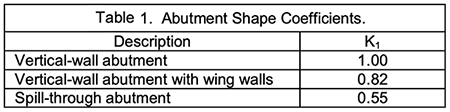online_scour_froehlich1989:  Calculation of abutment scour using the Froehlich 1989 equationFormula:

Fr = v /(gya)1/2

(ys / ya) = 2.27 K1 K2 (L' / ya)0.43 Fr 0.61 + 1

[ SI Units (metric):  ys, ya and L' in m ]
[ U.S. Customary Units:  ys, ya and L' in ft ]
[ Fr = Froude number; g = gravitational acceleration ]

Reference:

DESCRIPTION      [Sample Input]     [Main page]

To determine the abutment scour depth ys, the calculator requires the following information:

• Average depth of flow on the floodplain ya, in m (or ft).

• Mean flow velocity directly upstream of the embankment v1, in m/s (or fps).

• Length of active flow obstructed by the embankment L' , in m (or ft).

• Angle of embankment with flow θ , in m (or ft).

• The abutment shape (Figure 1).Fig. 1  Abutment shapes.

1. According to the shape of the abutment, define the coefficient of abutment K1, using Table 1.2. To determine the value of the coefficient for angle of embankment to flow K2, use the following equation: K2 = (θ/90)0.13

θ < 90o if embankment points downstream

θ > 90o if embankment points upstream

3. Calculate the Froude Number Fr for the given data using the equation Fr = v/(g.ya)1/2

4. Use the following equation to calculate the scour depth ys:

(ys / ya) = 2.27 K1 K2 (L' / ya)0.43 Fr 0.61 + 1

ya = Given

K1 = Calculated in step 1

K2 = Calculated in step 2

L' = Given

Fr = Calculated in step 3